# Dimensioning (2D)

The dimensioning functions can be used to dimension a parallel projection. Base dimensioning can be used to dimension the distance between two points, in a parallel projection.

Select an element

• Line
• Start and end point of the dimension
• Line and point
• Two points
• Arc or a circle
• Point and an arc of the circle
• Two arcs

Dimension associativity to an element

The dimension is an associative element that you select when adding a dimension.

If the element is removed, the dimensions associative to element is lost.

Dimension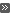Dimensioning of a Drawing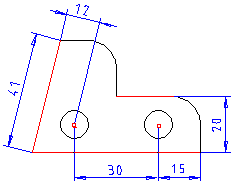There are three different dimensioning directions to dimension slanting lines: direction of the line, direction of the Y axis and direction of the X axis. The dimensioning direction is determined by the movement of the cursor before the position of the dimension has been selected.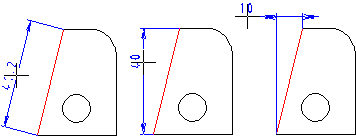There are two alternatives to diameter dimensions, depending on the position of the cursor before the position of the dimension has been selected.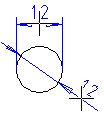Dimensioning can also be carried out as inch dimensioning.

Dimension as a Geometric Constraint

A dimension can act as a geometric constraint, if 2D Constraint Manager is enabled.

A drawing's dimensions added using the dimensioning function are updated when changes are made to geometry.

Vertex G4: They act as constraints in the drawing, if the Dimensioning tools make constraints check box has been selected on the Others tab of the Dimensions> Settings dialog box. The requirement is that the dimensioned element is one of the following: line, dimension start and end points, line and a point, or two lines.Miscellaneous dimensioning settings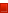Dimensions added to intersections or center points of lines are not updated when the geometry changes, and cannot act as constraints. Dimensions added with the Set dimension for flattened sheets dimensioning functions cannot act as geometric constraints.

Dimension types

The following dimension types to dimension parallel projection in the drawing:

• Dimension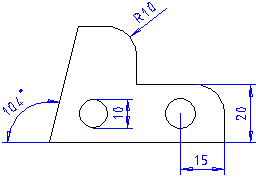Dimension

• Chain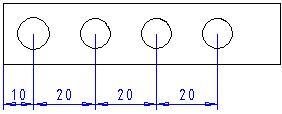Chain

• Baseline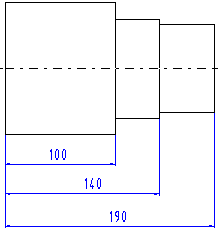Baseline

• Continuous baseline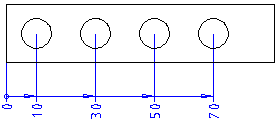Continuous baseline

• Diameter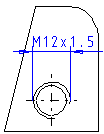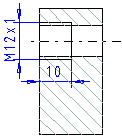Diameter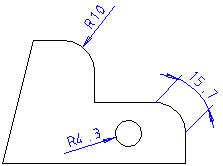Radius

• Arc lengthArc Length
• AngleDimension an Angle
• Flattening, vertical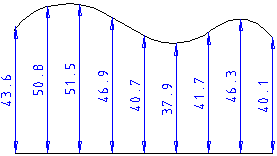Dimensioning Flattening, Vertical

• Dimensioning Flattening, Angle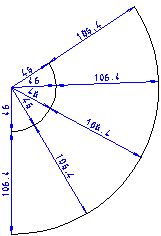Dimensioning Flattening, Angle